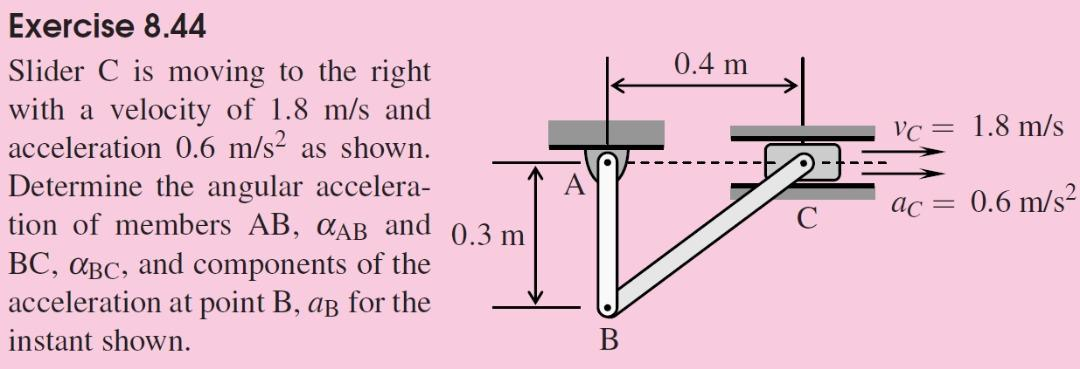# Question 0.4 m Vc = 1.8 m/s Exercise 8.44 Slider C is moving to the right with a velocity of 1.8 m/s and acceleration 0.6 m/s2 as shown. Determine the angular accelera- tion of members AB, CAB and 0.3 m BC, ABC, and components of the acceleration at point B, as for the instant shown. ac = 0.6 m/s2 BHMILVU The Asker · Mechanical EngineeringTranscribed Image Text: 0.4 m Vc = 1.8 m/s Exercise 8.44 Slider C is moving to the right with a velocity of 1.8 m/s and acceleration 0.6 m/s2 as shown. Determine the angular accelera- tion of members AB, CAB and 0.3 m BC, ABC, and components of the acceleration at point B, as for the instant shown. ac = 0.6 m/s2 B
More
Transcribed Image Text: 0.4 m Vc = 1.8 m/s Exercise 8.44 Slider C is moving to the right with a velocity of 1.8 m/s and acceleration 0.6 m/s2 as shown. Determine the angular accelera- tion of members AB, CAB and 0.3 m BC, ABC, and components of the acceleration at point B, as for the instant shown. ac = 0.6 m/s2 B